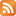F1 = 1

F2 = 1

F3 = F2 + F1 = 1 + 1 = 2

F4 = F3 + F2 = 2 + 1 = 3

F5 = F4 + F3 = 3 + 2 = 5

F6 = F6-1 + F6-2 = F5 + F4 = 5 + 3 = 8

F7 = F7-1 + F7-2 = 8 + 5 = 13

……

……

……

Fn = Fn-1 + Fn-2

## 如何求斐波那契数列的前十项之和

1 + 1 + 2 + 3 + 5 + 8 + 13 + 21 + 34 + 55 = 143

2,2,4,6,10,16,26,42,68,110

3,3,6,9,15,24,39,63,102,165

3 + 3 + 6 + 9 + 15 + 24 + 39 + 63 + 102 + 165 = 429

143/11 = 13

286/11 = 26

429/11 = 39

143 = 11 × 13 = 11 × 13 × 1

286 = 11 × 26 = 11 × 13 × 2

429 = 11 × 39 = 11 × 13 × 3

11×13×1

11×13×2

11×13×3

11×13×4

……

……

……

11×13×4

11 × 13 × n

n = 2 是以 2 和 2 开头的那个1. ### 动量和碰撞

22 年 9 月 12 日 07:19动量与碰撞之间的关系用动量守恒定律和许多现实生活中的例子清楚地解释了。

阅读更多

1. 单击下面的 HTML 链接代码。